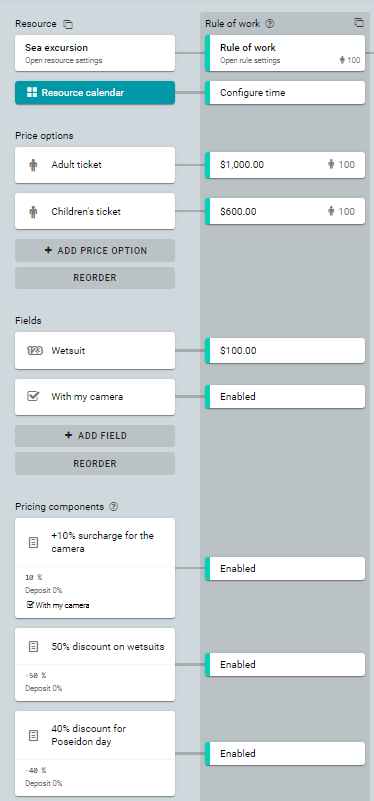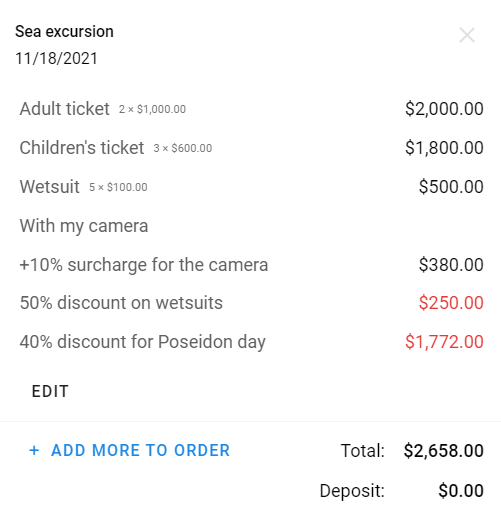arrow_back_iosQuestions
help

# How are the pricing components calculated in detail?

check_circle
You can use several discounts and surcharges at the same time. They are always calculated in order of their position in the configuration, from top to bottom.
Let's look at the calculation in details using an example. Let's say we have a sea tour:• 2 tickets for adults = 2 * 1000 = \$2000.
• 3 tickets for children = 3 * 600 = \$1800.
• 5 extra wetsuits = 5 * 100 = \$500.
• With his camera
In this case, the pricing components are applied in the following order:
• 10% surcharge on the camera price
• 50% discount on wetsuits
• 40% holiday discount on the total cost.
Before we will apply the discounts, we have this table of calculation:
 Element Cost Calculation Adult ticket 2000 2000 Children's ticket 1800 1800 Wetsuit 500 500

## 10% surcharge on the camera price

For this price component, only price options are used in calculation. This means that the cost of the wetsuit is not taken into account when calculating the surcharge.
We calculate. 10% * (2000 + 1800) = \$380.
The surcharge is distributed evenly between price options. For \$190 each.
 Element Cost Calculation Adult ticket 2000 2190 Children's ticket 1800 1990 Wetsuit 500 500 10% surcharge 380 -

## 50% discount on wetsuits

For this price component, conversely, only the wetsuit is used in calculation. This means that the cost of the price options is not taken into account when calculating this surcharge.
Let's calculate: 50% * (500) = \$250.
Discount applies only to the wetsuit.
 Element Cost Calculation Adult ticket 2000 2190 Children's ticket 1800 1990 Wetsuits 500 250 10% surcharge 380 - 50% discount -250 -

## 40% discount on the total cost

Discounts and surcharges are calculated from the Calculation field (not from the initial cost). This discount applies to all price options and fields. It needs to be distributed evenly among all these elements.
40% * (2190 + 1990 + 250) = \$1772.
= 590.67 + 590.67 + 590.67
You need to subtract \$590.67 from each element.
We start subtracting from the smallest elements.
Wetsuit: 250 - 590.67 = -\$340.67.
It turned out that the wetsuit price was overlapped completely, and there are unspent funds left. Let's recalculate the discount taking into account these funds.
590.67 + 590.67 + 340.67 = \$1522.
= 761 + 761
This discount should be distributed between the two remaining items.
Children's ticket: 1990 - 761 = \$1229.
Adult ticket: 2190 - 761 = \$1429.
 Element Cost Calculation Adult ticket 2000 1429 Children's ticket 1800 1229 Wetsuit 500 0 10% surcharge 380 - 50% discount -250 - 40% discount -1772 - Amount 2658 2658
As a result, the invoice displays all the information from the second column. The calculations are hidden.